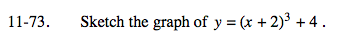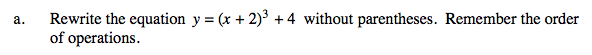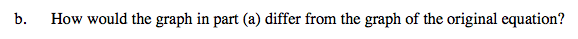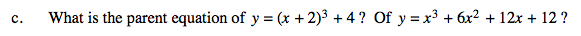Home > A2C > Chapter 11 > Lesson 11.2.3 > Problem11-73

11-73.
1. Sketch the graph of y = (x + 2)3 + 4. Homework Help ✎

1. Rewrite the equation y = (x + 2)3 + 4 without parentheses. Remember the order of operations.

2. How would the graph in part (a) differ from the graph of the original equation?

3. What is the parent equation of y = (x + 2)3 + 4? Of y = x3 + 6x2 + 12x + 12?y = x3 + 6x2 + 12x + 12The equations are equivalent. Their graphs do not differ.What is the highest power in each?